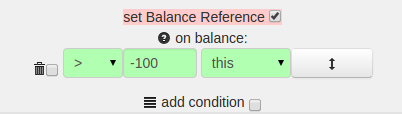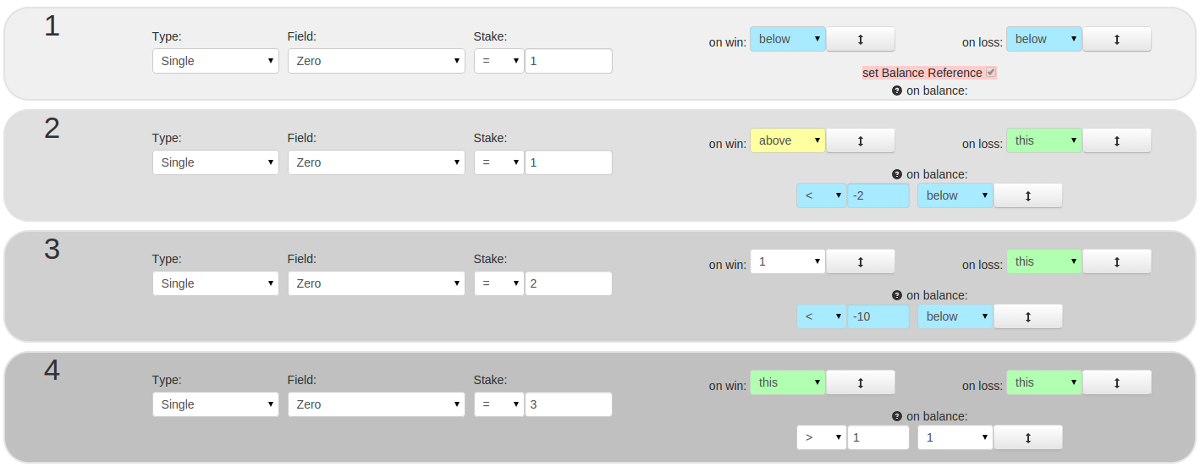adminyouroul.com0 180

The balance function is activated by adding a condition.
After confirmation by the click on update, the configuration of the condition appears directly beneath.

The compared balance is the difference, calculated from the current balance and the balance reference:
Balance = current balance - balance reference
If the balance reference is never reset then the following applies:
Balance = current balance (-0)

If set is enabled, the balance reference gets the value of the current balance, after the evaluation of the respective step.
Is set of the balance reference not activated in any step then the value always remains 0.

Applies a set condition, so is on balance prior to on win / on loss and on field win.
If there are several conditions in one step the uppermost fulfilled condition comes to application.
Priority: from top to bottom, top firstAn example roulette strategy: on balance1st step:
Bet 1 piece on Zero.
On win and on loss of this bet, jump to step 2. (here, in every case)
The function "on balance" is not activated.
The balance reference is set. After the evaluation of this step, the amount of balance is remembered as balance reference.

2nd step:
Bet 1 piece on Zero.
On loss repeat step 2.

The function "on balance" is activated:
After the evaluation of the step, the condition "balance < -2" is checked:
Assuming the first step was lost, the balance reference would be -1.
When the second step was lost also, the balance would then be -2.
The condition: "current balance < balance reference -2" would be: -2 < -1 -2 means: -2 < -3.
In this case the condition is not true, the jump of "on loss" would be made.

The jumps of "on win" and "on loss" are subordinated in that case.

3rd step:
Bet 2 pieces on Zero.
On loss repeat step 3.

The function "on balance" is activated:
After the evaluation of the step, the condition "balance < -10" is checked.
If the condition is true, the jump to step 4 is made - prior to "on win" / "on loss".

4th step:
Bet 3 pieces on Zero.
On win and on loss repeat step 4.

The function "on balance" is activated:
After the evaluation of the step, the condition "balance > 1" is checked.
If the condition is true, the jump to step 1 is made - prior to "on win" / "on loss".

You can see the functionality in the public tests of this strategy.